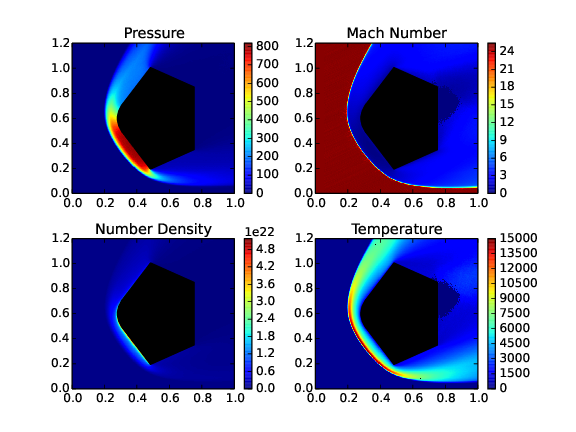# Research

I am interested in the development of computational models and studying problems related gas dynamics: both conventional and rarefied. I have experience in a broad range of methods from solving Schrodinger equation based Computational Chemistry algorithms to Molecular Dynamics simulations to Rarefied Gas Dynamics (Nonlinear Coupled Constitutive Relation (NCCR) and Direct Simulation Monte Carlo (DSMC)) to conventional Computational Gas Dynamics.

## Molecular Dynamics based Modelling

The overall ideology of this is to understand complex gas physics phenomena at a molecular level. The data obtained can be used to propose better models for either continuum based NSF equations or the DSMC method. The benefit of such a fundamental approach is the extensibility and customization in various computational frameworks for a wide range of interdisciplinary applications.

### Ab-initio Chemical Reaction Modelling

An improved chemical reaction model that employs reactive cross-section databases that are generated using the ab-initio methods is a better alternative than the phenomenological models. Reactive cross-sections are a measure of how likely an interaction at the molecular level will lead to a reaction. The development included the generation of Potential Energy Surfaces (PES) that are based on computational chemistry algorithms and generation of reactive cross-section databases using the Quasi-Classical Trajectory method. This cross-section database was used to model an alternative method of simulating chemical reactions in DSMC.

The cross-section databases generated have applications in simulation techniques other than the DSMC method. Improved two-temperature models: Nonequilibrium total temperature (NETT) and Nonequilibrium piece-wise interpolation (NEPI) model, are based on the reactive cross-section database and developed for PDE based framework (NSF equations).

### Ab-initio Inelastic Energy Exchange Model

Molecular dynamics simulations can also be used to calculate the inelastic collision cross-section. In the DSMC framework, Larsen-Borgnakke (LB) model is a popular model for simulating inelastic energy exchange. The parameters used in the LB model utilize the values of macroscopic quantities such as the translational temperature, pressure and number density. In the case of flows with a high degree of non-equilibrium, the definition of macroscopic quantities such as the temperature is questionable. Although the LB model handles inelastic collisions reasonably well in most cases; it may not be appropriate to use this model in case of flows with a high degree of non-equilibrium. I am currently working on this area. State-to-state inelastic cross-sections using molecular simulations though computationally expensive will indeed be a better alternative to the LB model. The generation of such a database will require ingenuity to circumvent massive computational demands.

### Ab-initio Collision Cross-section Model and non-equilibrium Transport Properties

Generally, the total collision cross-sections are assumed to be a function of relative translational energy only. In the DSMC framework, several models such as the Variable Hard Sphere (VHS) and Variable Soft Sphere (VSS) models are based on this idea. The validity of this assumption is questionable when simulating flows with diatomic and polyatomic gases.

A new collision model is developed to address the shortcomings of the prevalent models. The new model uses the molecular simulations to obtain the total collision cross-section as a function of translational energy and ro-vibrational levels making it suitable for flows with polyatomic gases. It is observed that the vibrational levels in the case of the diatomic molecules have a crucial impact on the scattering and subsequently on the total collision cross-sections. The next goal of this project is to propose a simple collision model based on this database for the DSMC framework that can potentially supersede the phenomenological models.

The total collision cross-sections are fundamental in calculating transport coefficients from a theoretical point of view. The viscosity-temperature and conductivity-temperature relations are well established. However, these fits assume equilibrium conditions between the different mode of energies. It is observed from the ongoing study that the vibrational temperature has a distinct effect on the transport coefficients when compared to the translational temperature. This implies transport properties are not equilibrium properties and can result in improper understanding of flows with high degree of non-equilibrium (such as the hypersonic flows).

## Rarefied Gas Dynamics

### Hypersonic Re-entry Flows

Simulating flow around the re-entry vehicles is challenging as the high energy in the post-shock region leads to various interesting phenomena such as inelastic energy exchange, chemical and ionization reactions, thermal and chemical surface ablation and radiative heat transfer. Further, the flow contains a high degree of non-equilibrium.Figure: Typical flow physics around a re-entry vehicle at non-zero angle of attack

### DSMC Direct Simulation Monte Carlo

Direct Simulation Monte Carlo (DSMC) is a particle method that is employed to study high Knudsen number flows. In DSMC, every simulated particle represents a large number of real particles. Generally, the time step chosen should be a fraction of the local mean collision time. Although the method is Lagrangian in nature, the simulation domain is divided into a mesh of cells with a constraint that the size of these cells does not exceed the local mean free path. The rectilinear motion of the particles is followed by cell-wise probabilistic collisions among the resident particles of the cells. The cell restricts the choice of collision partners in a way similar to the cutoff radius or radius of influence in other particle methods such as molecular dynamics or smoothed particle hydrodynamics.